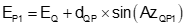### B. Tangent line-arc intersection

The next two solutions deal with tangent a tangent line and arc.

Known information

• Coordinates at point Q
• Direction from point Q
• Coordinates of radius point, point O

Determine

• Coordinates of tangent point PFigure I-2 Radius determination

Solution process

Inverse along the line from point Q to the radius point, O, to determine the direction and length of the line.

Compute angle Q as the difference between the directions AzQO and AzQP, subtracting the smaller from the larger, depending on which side of line QO line QP is.

Solve the following equationsEquation I-8Equation I-9Equation I-10Equation I-11

Known information

• Coordinates of radius point, point O
• Coordinates of point Q

Determine

• Direction of a line from point Q tangent to the arc; there are two possible directions, compute both
• Coordinates of the two tangent points P1 and P2

Determine the direction of a line tangent to an arc.Figure I-3 Tangent direction determination

Solution process

Inverse along the line from point point Q to the radius point O to determine its direction and length.

Compute angle g using Equation I-12.Equation I-12

Angle g is used with the direction of line point Q to radius point O to determine the direction from point Q to each intersection point.

The distance from point Q to each intersection point is:Equation I-13

To compute the coordinates of P1, perform a Forward Computation from point Q:Equation I-13Equation I-14Equation I-15

To compute the coordinates of point P2, perform a Forward Computation from  point Q:Equation I-16Equation I-17Equation I-18